# Gold, silver, bronze

How many ways can we divide gold, silver, bronze medails if there are 6 people competing?

Correct result:

n =  120

#### Solution:

$n=6 \cdot \ 5 \cdot \ 4=120$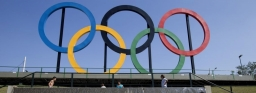We would be pleased if you find an error in the word problem, spelling mistakes, or inaccuracies and send it to us. Thank you!Tips to related online calculators
Would you like to compute count of combinations?

## Next similar math problems:There are 15 boys and 12 girls at the graduation party. Determine how many four couples can be selected.
• DivideHow many different ways can three people divide 7 pears and 5 apples?
• Combinations of sweatersI have 4 sweaters two are white, 1 red and 1 green. How many ways can this done?
• Sweater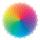Dana confuses sweater and wool has a choice of seven colors. In how many ways she can choose from three colors to the sleeves?
• Phone numbersHow many 7-digit telephone numbers can be compiled from the digits 0,1,2,..,8,9 that no digit is repeated?
• Three-digit numbersHow many three-digit numbers are from the numbers 0 2 4 6 8 (with/without repetition)?
• Math logicThere are 20 children in the group, each two children have a different name. Alena and John are among them. How many ways can we choose 8 children to be among the selected A) was John B) was John and Alena C) at least one was Alena, John D) maximum one wa
• Five letters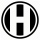How many ways can five letters be arranged?
• Peaches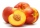There are 20 peaches in the pocket. 3 peaches are rotten. What is the probability that one of the randomly picked two peaches will be just one rotten?
• Boys and girlsThere are 20 boys and 10 girls in the class. How many different dance pairs can we make of them?
• The manufacturer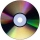The manufacturer found that 3% of the plates produced had a malfunction. Of the compliant, 75% are first and 25% second. What is the probability of producing first and second class plates?
• Shelf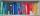How many ways are there to arrange 6 books on a shelf?
• SalamiHow many ways can we choose 5 pcs of salami if we have 6 types of salami for 10 pieces and one type for 4 pieces?
• Sum or productWhat is the probability that two dice fall will have the sum 7 or product 12?
• Glass with icecream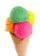We have 6 kinds of ice cream and 5 kinds of fruit. We put 3 cups of ice cream and 2 fruits into each glass. How many can unique decorated glasses be?
• MedalsIn how many ways can be divided gold, silver and bronze medal among 21 contestant?
• The camp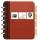At the end of the camp a 8 friends exchanged addresses. Any friend gave remaining 7 friends his card. How many addresses they exchanged?